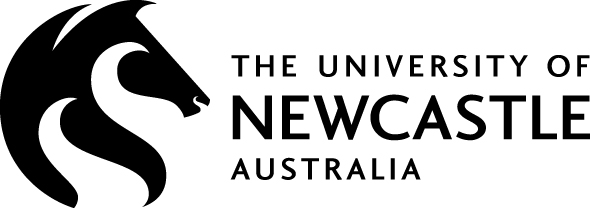# Research Group — Zero-Dimensional Symmetry

Date Time Room Speaker Title
04.11.2019 10.00 V 108 Max Carter Project Presentation Rehearsal

29.07.2019 11.00 MC LG17 George Willis Computing in automorphism groups of trees

20.05.2019 13.00 W 243 Ben Brawn Automorphisms of forests of quasi-label-regular rooted trees
We investigate when the automorphism group of a quasi-label-regular rooted tree (QLRRT) is trivial or non-trival. We determine when a QLRRT has a finite domain and use this to write its automorphism group as an iterated wreath product. When a QLRRT doesn't have a finite domain, sometimes we can still write its automorphism group as an iterated wreath product and other times we are unlucky and need some number of coupled self-referential equations to describe the group.

08.05.2019 10.00 W 243 Ben Brawn Forests of quasi-label-regular rooted trees and their almost isomorphism classes
We introduce almost isomorphisms of locally-finite graphs and in particular, trees. We introduce a type of infinite tree, dubbed label-regular, and consider trees that are label-regular except at a finite number of vertices, which we call quasi-label-regular trees. We show how to determine if two quasi-label-regular trees are almost isomorphic or not. We count the number of equivalence classes of quasi-label-regular trees under almost isomorphisms and find this number ranges from finite to infinite. When there are a finite number we show how to determine it and suggest a way to choose representatives for the equivalence classes.

27.03.2019 10.00 W 243 Yossi Bokor What doughnuts tell us about data
The old joke is that a topologist can't distinguish between a coffee cup and a doughnut. A recent variant of Homology, called Persistent Homology, can be used in data analysis to understand the shape of data. I will give an introduction to persistent Homology and describe two example applications of this tool.

05.02.2019 12.00 MC G29 Alastair Anderberg, Max Carter, Peter Groenhout
William Roland-Batty, Chloe Wilkins
Summer Projects Dress Rehearsal

18.12.2018 14.00 MC LG17 Max Carter, Peter Groenhout Summer Projects

11.12.2018 14.00 MC G29 Davide Spriano Convexity and generalization of hyperbolicity
Almost by definition, the main tool and goal of Geometric Group Theory is to find and exploit correspondences between geometric and algebraic features of groups. Following this philosophy, I will focus on the question: what does it mean for a sub(space/group) to "sit nicely" inside a bigger (space/group)? Focusing on groups, for a subgroup H of a group G, possible answers for the above question are when the subgroup H is: quasi-isometrically embedded, undistorted, normal/malnormal, finitely generated, geometrically separated...
Many of the above are equivalent when H is a quasiconvex subgroup of a hyperbolic group G, providing very successful correspondences between geometric and algebraic properties of subgroups.
The goal of this talk is to review quasiconvexity in hyperbolic spaces and try to generalize several of those features in a broader setting, namely the class of hierarchically hyperbolic groups (HHG). This is a joint work with Hung C. Tran and Jacob Russell.

04.12.2018 all week Adelaide AustMS Meeting

27.11.2018 14.00 MC LG17 Alejandra Garrido Hausdorff dimension and normal subgroups of free-like pro-$p$ groups
Hausdorff dimension has become a standard tool to measure the "size" of fractals in real space. However, it can be defined on any metric space and therefore can be used to measure the "size" of subgroups of, say, pro-$p$ groups (with respect to a chosen metric). This line of investigation was started 20 years ago by Barnea and Shalev, who showed that $p$-adic analytic groups do not have any "fractal" subgroups, and asked whether this characterises them among finitely generated pro-$p$ groups.
I will explain what all of this means and report on joint work with Oihana Garaialde and Benjamin Klopsch in which, while trying to solve this problem, we ended up showing an analogue of a theorem of Schreier in the context of pro-$p$ groups of positive rank gradient: any finitely generated infinite normal subgroup of a pro-$p$ group of positive rank gradient is of finite index. I will also explain what "positive rank gradient" means, and why pro-$p$ groups with such a property are "free-like".

20.11.2018 14.00 MC 110 Thibaut Dumont Cocycles on trees and piecewise translation action on locally compact groups
In the first part of this seminar, I will present some geometric cocycles associated to trees and ways to compute their norms. Similar construction exists for Euclidean buildings but no satisfactory estimates of the norm is currently known. In the second part, I will discuss some ongoing research with Thibaut Pillon on actions the infinite cyclic group by piecewise translations on locally compact group. Piecewise translation actions have been well studied for finitely generated groups, e.g. by Whyte, and provide positive answers to the von-Neumann-Day problem or the Burnside problem. The generalization to LC-groups was introduced by Schneider. The topic seems to have interesting implications for tdlc-groups.

13.11.2018 all day X 602 EViMS Workshop

12.11.2018 14.00 MC G29 Anne Thomas Divergence in right-angled Coxeter groups
The divergence of a pair of geodesics in a metric space measures how fast they spread apart. For example, in Euclidean space all pairs of geodesics diverge linearly, while in hyperbolic space all pairs of geodesics diverge exponentially. In the 1980s Gromov proved that in symmetric spaces of non-compact type, the only possible divergence rates are linear or exponential, and he asked whether the same dichotomy holds in CAT(0) spaces. Soon afterwards, Gersten used these ideas to define a quasi-isometry invariant, also called divergence, which measures the "worst" rate of divergence. Gersten and others have since found many examples of finitely generated groups with quadratic divergence. We study divergence in right-angled Coxeter groups with triangle-free defining graphs. Using the structure of certain flats in the associated Davis complex, which is a CAT(0) square complex, we characterise such groups with linear and quadratic divergence, and construct examples of right-angled Coxeter groups with divergence polynomial of arbitrary degree. This is joint work with Pallavi Dani (Louisiana State University).

06.11.2018 all day U Sydney Group Actions Seminar held at the University of Sydney

30.10.2018 14.00 MC LG17 Reading Group

23.10.2018 all day U Sydney Group Actions Seminar held at the University of Sydney

16.10.2018 14.00 MC LG17 Alejandra Garrido Maximal subgroups of some groups of intermediate growth
Given a group one of the most natural things one can study about it is its subgroup lattice, and the maximal subgroups take a prominent role. If the group is infinite, one can ask whether all maximal subgroups have finite index or whether there are some (and how many) of infinite index. After telling some historical developments on this question, I will motivate the study of maximal subgroups of groups of intermediate growth and report on joint work with Dominik Francoeur where we give a complete description of all maximal subgroups of some "siblings" of Grigorchuk's group.

09.10.2018 14.00 MC LG17 Dave Robertson Algebraic theory of self-similar groups
I will describe the relationship between self-similar groups, permutational bimodules and virtual group endomorphisms. Based on chapter 2 of Nekrashevych’s book.

02.10.2018 14.00 MC LG17 Alex Bishop The Group Co-Word Problem
In this talk, we will introduce a class of tree automorphism groups known as bounded automata. From this definition, we will see that many of the interesting examples of self-similar groups in the literature are members of this class.
A problem in group theory is classifying groups based on the difficulty of solving their co-word problems, that is, classifying them by the computational difficulty to decide if a word is not equivalent to the identity. Some well-known results in this study are that a group has a co-word problem given by a regular language if and only if it is finite, a deterministic context-free language if and only if it is virtually free, and a deterministic one-counter machine if and only if it is virtually cyclic. Each of these language classes corresponds to a natural and well-studied model of computation.
We will show that the class of bounded automata groups has a co-word problem given by an ET0L language – a class of formal language which has recently gained popularity in areas of group theory. This strengthens a recent result of Holt and Röver (who showed this result for a less restrictive class of language) and extends a result of Ciobanu-Elder-Ferov (who proved this result for the first Grigorchuk group).

25.09.2018 14.00 MC LG17 Timothy Bywaters Spaces at infinity for hyperbolic totally disconnected locally compact groups
Every compactly generated t.d.l.c. group acts vertex transitively on a locally finite graph with compact open vertex stabilisers. Such a graph is called a rough Cayley graph and, up to quasi-isometry, is an invariant for the group. This allows us to define Gromov hyperbolic t.d.l.c. groups and their Gromov boundary in a way analogous to the finitely generated case. The space of directions of a t.d.l.c. group is a metric space 'at infinity' obtained by analysing the action of the group on the set of compact open subgroups. It is particularly useful for detecting flat subgroups, think subgroups that look like $\mathbb{Z}^n$.
In my talk, I will introduce these two concepts of boundary and give some new results which relate them. Time permitting, I may also give details about the proofs.

10.09.2018 14.00 MC G29 Colin Reid Endomorphisms of profinite groups
Given a profinite group $G$, we can consider the semigroup $\mathrm{End}(G)$ of continuous homomorphisms from $G$ to itself. In general $\lambda \in\mathrm{End}(G)$ can be injective but not surjective, or vice versa: consider for instance the case when $G$ is the group $F_p[[t]$ of formal power series over a finite field, $n$ is an integer, and $\lambda_n$ is the continuous endomorphism that sends $t^k$ to $t^{k+n}$ if $k+n \ge 0$ and $0$ otherwise. However, when $G$ has only finitely many open subgroups of each index (for instance, if $G$ is finitely generated), the structure of endomorphisms is much more restricted: given $\lambda \in\mathrm{End}(G)$, then $G$ can be written as a semidirect product $N \rtimes H$ of closed subgroups, where $\lambda$ acts as an automorphism on $H$ and a contracting endomorphism on $N$. When $\lambda$ is open and injective, the structure of $N$ can be restricted further using results of Glöckner and Willis (including the very recent progress that George told us about a few weeks ago). This puts some restrictions on the profinite groups that can appear as a '$V_+$' group for an automorphism of a t.d.l.c. group.

03.09.2018 14.00 MC G29 Stephan Tornier An introduction to self-similar groups
We introduce the notion of self-similarity for groups acting on regular rooted trees as well as their description using automata and wreath iteration. Following the definition of Grigorchuk's group we show that it is an infinite, finitely generated $2$-group. The proof illustrates the use of self-similarity.

27.08.2018 14.00 MC G29 George Willis The tree representation theorem and automorphism groups of rooted trees
(joint work with R. Grigorchuk ad D. Horadam) The tree representation theorem represents a certain group associated with the scale of an automorphism of a t.d.l.c. group as acting by symmetries of a regular (unrooted) tree. It shows that groups acting on regular trees are a fundamental part of the theory of t.d.l.c. groups.
There is also an extensive theory of self-similar and self-replicating groups of symmetries of rooted trees which has developed from the discovery (or creation) of examples such as the Grigorchuk groups.
It will be seen in this talk that these two branches of research are studying essentially the same groups.

20.08.2018 14.00 MC G29 George Willis Locally pro-p contraction groups are nilpotent
A contraction group is a pair $(G,\alpha)$ in which $G$ is a locally compact group and $\alpha$ is an automorphism of $G$ such that $\alpha^n(x)\to 1$ as $n\to\infty$. In joint work with H. Glöckner, it is shown that every contraction group is the direct sum of closed subgroups $$G = D\oplus T$$ with $D$ divisible, i.e. for every $x\in D$ and $n>0$ there is $y\in D$ with $y^n =x$ and $T$ torsion, i.e. there is $n>0$ such that $x^n = 1$ for every $x\in T$. Furthermore, $D$ is the direct sum $$D = \bigoplus_{i=1}^k D_{p_i}$$ of $p_i$-adic analytic nilpotent contraction groups for some prime numbers $p_1,\ldots, p_k$. The torsion subgrou $T$ may also be written as a composition series of simple contraction groups. In the case when all the composition factors are of the form $\mathbb{F}_p(\!(t)\!), \alpha$ with $\alpha$ being the automorphism of multiplication by $p$, it follows easily that $G$ is a solvable group. These ideas will be explained in the talk and a sketch will be presented of a proof that $G$ is in fact nilpotent in this case.

13.08.2018 14.00 MC G29 Michal Ferov Separating cyclic subgroups in graph products of groups
(joint work with Federico Berlai) A natural way to study infinite groups is via looking at their finite quotients. A subset S of a group G is then said to be (finitely) separable in G if we can recognise it in some finite quotient of G, meaning that for every g outside of S there is a finite quotient of G such that the image of g under the canonical projection does not belong to the image of S. We can then describe classes of groups by specifying which types of subsets do we require to be separable: residually finite groups have separable singletons, conjugacy separable groups have separable conjugacy classes of elements, cyclic subgroup separable groups have separable cyclic subgroups and so on... We could also restrict our attention only to some class of quotients, such as finite p-groups, solvable, alternating... Properties of this type are called separability properties. In case when the class of admissible quotients has reasonable closure properties we can use topological methods.
We prove that the property of being cyclic subgroup separable, that is having all cyclic subgroups closed in the profinite topology, is preserved under forming graph products.
Furthermore, we develop the tools to study the analogous question in the pro-p case. For a wide class of groups we show that the relevant cyclic subgroups - which are called p-isolated - are closed in the pro-p topology of the graph product. In particular, we show that every p-isolated cyclic subgroup of a right-angled Artin group is closed in the pro-p topology and, consequently, we show that maximal cyclic subgroups of a right-angled Artin group are p-separable for every p.

06.08.2018 14.00 MC G29 Stephan Tornier Totally disconnected, locally compact groups from transcendental field extensions
(joint work with Timothy Bywaters) Let E over K be field extension. Then the group of automorphisms of E which pointwise fix K is totally disconnected Hausdorff when equipped with the permutation topology. We study examples, aiming to establish criteria for this group to be locally compact, non-discrete and compactly generated.

30.07.2018 14.00 MC G29 Ben Brawn Voltage and derived graphs and their relation to the free product of graphs
We look at a classical construction known as ordinary voltage graphs and their derived graphs. We show how to construct the free product of graphs as the derived graph of a voltage graph whose base graph is the Cartesian product of the given graphs with a specific voltage assignment. We find that the voltage group is always a free group and give the number of generators needed.

23.07.2018 14.00 MC G29 Colin Reid A lemma for group actions on zero-dimensional spaces
I present a lemma concerning a group action on a locally compact zero-dimensional spaces, where the group has a 'small' (compact, say) generating set, relating invariant compact sets with orbit closures. A typical example to have in mind is a compactly generated tdlc group acting on itself by conjugation, where we use conditions on closures of conjugacy classes to deduce the existence of compact normal subgroups. The idea of the lemma has appeared several times in the literature but does not appear to have been given explicitly in this form. I will discuss various applications depending on time.

12.06.2018 14.00 V 206 Dave Robertson Topological full groups - Part II
For an action of a group G on the Cantor set, we can construct a group of transformations of the Cantor set that are constructed 'piecewise' from elements of G. This is called the topological full group of G. Examples include the topological full groups associated to a minimal homeomorphism of the Cantor set considered by Giordano, Putnam and Skau, and Neretin's group of spheromorphisms. I will describe the construction using groupoids, and show how certain examples admit a totally disconnected locally compact topology. This is based on work in progress with Alejandra Garrido and Colin Reid.

06.06.2018 14.00 V 206 George Willis Free products of graphs - Part II

05.06.2018 14.00 V 206 Dave Robertson Topological full groups - Part I
For an action of a group G on the Cantor set, we can construct a group of transformations of the Cantor set that are constructed 'piecewise' from elements of G. This is called the topological full group of G. Examples include the topological full groups associated to a minimal homeomorphism of the Cantor set considered by Giordano, Putnam and Skau, and Neretin's group of spheromorphisms. I will describe the construction using groupoids, and show how certain examples admit a totally disconnected locally compact topology. This is based on work in progress with Alejandra Garrido and Colin Reid.

30.05.2018 14.00 V 206 George Willis Free products of graphs - Part I

29.05.2018 14.00 V 206 Michal Ferov Profinite words and inverse limits of finite state automata
In the case of finitely generated discrete groups, the problem of deciding whether a product of a sequence of generators and their inverses represents the trivial element is known as the word problem. Somewhat surprisingly, the complexity of word problem is tightly connected to the structure and geometry of the group: a classical result of Anisimov states that a group has word problem decidable by finite-state automaton if and only if the group is finite; similarly, result of Muller and Shupp states that a group has word problem is decidable by push-down automaton if and only if the group is virtually-free. In my talk, I will define inverse limits of finite-state automata and discuss how it might be useful for studying totally-disconnected locally-compact groups.

22.05.2018 14.00 V 206 Thomas Murray On automorphism groups of regular rooted groups
Starting with the automorphism group of a regular, locally finite tree the tree representation theorem leads us to groups acting on a regular, rooted tree. Furthermore these groups satisfy a property called R and are profinite. As a result, the study of these groups may be reduced to those that act on a finite depth regular rooted tree with corresponding finite version of property R. We introduce the idea of studying such groups with geometric objects in order to study trees of higher valency and investigate conjectures made for the binary rooted tree.

15.05.2018 14.00 LSTH 100 George Willis School Seminar — Zero-Dimensional Symmetry
The pleasure and utility of observing symmetry in nature may be found in the mathematics of symmetry, which is known as group theory. Zero-dimensional symmetry is the symmetry of networks and relationships, such as a family tree. In contrast, physical objects, such as a sphere, have positive-dimensional symmetry. While positive-dimensional symmetry has been well understood for more than a century (and is applied in physics) it is only in the last 25 years that our understanding of zero-dimensional symmetry has begun to catch up. Even though great progress is being made, we still aren’t sure how close we are to having the full picture.

08.05.2018 14.00 V 206 Thomas Taylor Automorphisms of Cayley graphs for right-angled Artin groups

01.05.2018 14.00 V 126 George Willis Project — Zero-Dimesional Symmetry
The project on 0-dimensional symmetry, that is, totally disconnected locally compact groups, is organised around four themes, namely, ‘Structure theory’, ‘Geometries’, ‘Local structure and commensurators’ and ‘Representations and computation’. These themes relate to the scale function on a t.d.l.c. group as follows. The scale itself is defined directly in terms of commensuration and the tidying procedure enables computation of the scale. Tidy subgroups can also be characterised geometrically, and the scale behaves naturally under structural decompositions of groups.

24.04.2018 14.00 V 205 Ben Brawn On quasi-label-regular trees and their classification
We introduce almost isomorphisms of locally-finite infinite graphs and in particular trees. We introduce a type of infinite tree, dubbed label-regular, and consider trees that are label-regular except at a finite number of vertices, which we call quasi-label-regular trees. We show how to determine if two quasi-label-regular trees are almost isomorphic or not. We count the number of equivalence classes of quasi-label-regular trees under almost isomorphisms and find this number ranges from finite to infinite.

17.04.2018 14.00 V 205 Stephan Tornier Groups acting on trees with non-trivial quasi-center
We highlight the role of the quasi-center of a t.d.l.c. group in Burger-Mozes theory and present new results concerning the types of automorphisms that the quasi-center of a non-discrete subgroup of the automorphism group of a regular tree may contain in terms of its local action. A theorem which shows that said result is sharp is also presented. We include a proof of the fact that a non-discrete, locally transitive subgroup of the automorphism group of a regular tree does not contain a quasi-central involution.

10.04.2018 14.00 V 205 Stephan Tornier An introduction to Burger-Mozes theory
We recall the types of automorphisms of trees and introduce the notion of local action. After an excursion into permutation group theory, specifically the notions of transitivity, semiprimitivity, quasiprimitivity, primitivity and 2-transitivity, we give an introduction to Burger-Mozes theory of closed, non-discrete subgroups of the automorphism group of a (regular) tree which are locally quasiprimitive.

05.04.2018 14.00 V 205 Colin Reid Totally disconnected, locally compact groups
I give an overview of totally disconnected, locally compact (t.d.l.c.) groups: what they are and in what contexts they arise. In particular, t.d.l.c. groups encompass many classes of automorphism groups of structures, and also occur as completions of groups that have commensurated subgroups. I then discuss some techniques and approaches for studying them, particularly with an eye to general structural questions, and the recent progress that has been made.
We investigate the structure of topological groups and, in particular, symmetry groups of discrete structures such as networks, combinatorial graphs and complexes. The topology on these symmetry groups is locally compact and totally disconnected; such spaces are also called 0-dimensional. The overall goal of our research is to achieve a complete description of 0-dimensional groups that says how a given group may be broken down into simpler ones; classifies the simple ones; relates the global structure of the groups to their local structure; and says how they may be represented geometrically, algebraically and computationally.

Our research may be contrasted with theory of connected locally compact groups, which was largely completed in the 1950s with the solution of Hilbert's Fifth Problem. Building on the earlier foundational work of Sophus Lie, Elie Cartan, Emmy Noether and Hermann Weyl, this theory was vital in twentieth century physics because 4-dimensional space-time, the equations of the quantum atom, and the strong nuclear force all have connected symmetry groups. Whereas they are symmetry groups of physical space, the totally disconnected groups we seek to understand are the symmetry groups of networks, or cyberspace.

Experimental calculations with finite groups guide many aspects of this research. Our longer term aim is to convert theorems into algorithms and to develop computational and visualisation tools for infinite groups which will be made available online. Since the groups are topological, using approximation to reduce the calculations to finite groups will be an important aspect of achieving that aim (p-adic analysis is a particular but restricted case where this approximation is understood).

0-dimensional groups have links with combinatorics, number theory, finite and profinite group theory, geometric group theory, Lie groups, harmonic analysis, descriptive set theory and logic. International research on 0-dimensional groups has grown rapidly in the past decade or so and much of this new activity has been stimulated by breakthroughs made at the University of Newcastle. Our researchers continue to make ground-breaking advances in collaboration with mathematicians from America, Europe and Asia. This research is being supported by Australian Research Council funds of $2.8 million in the period 2018-22.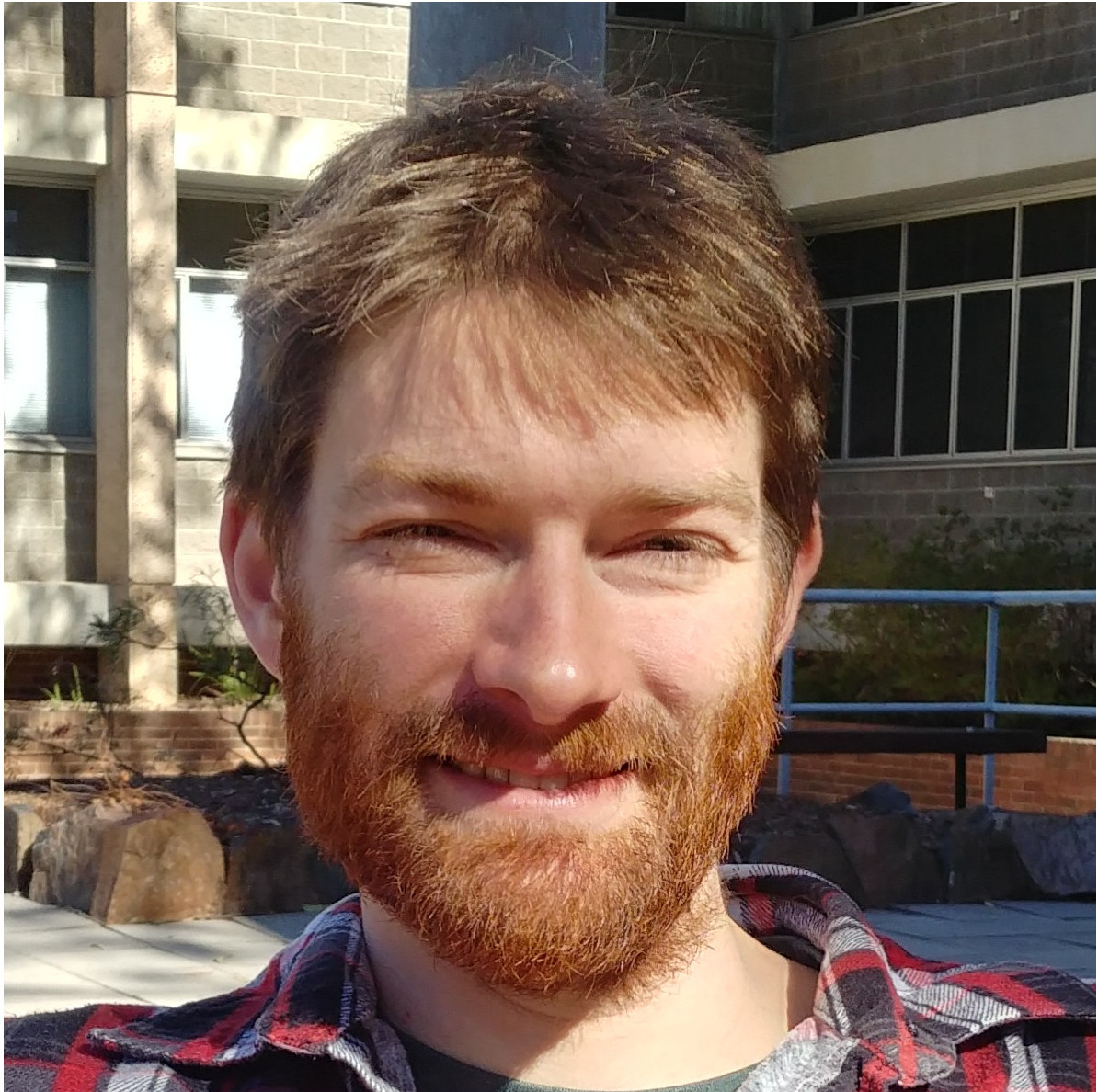Ben Brawn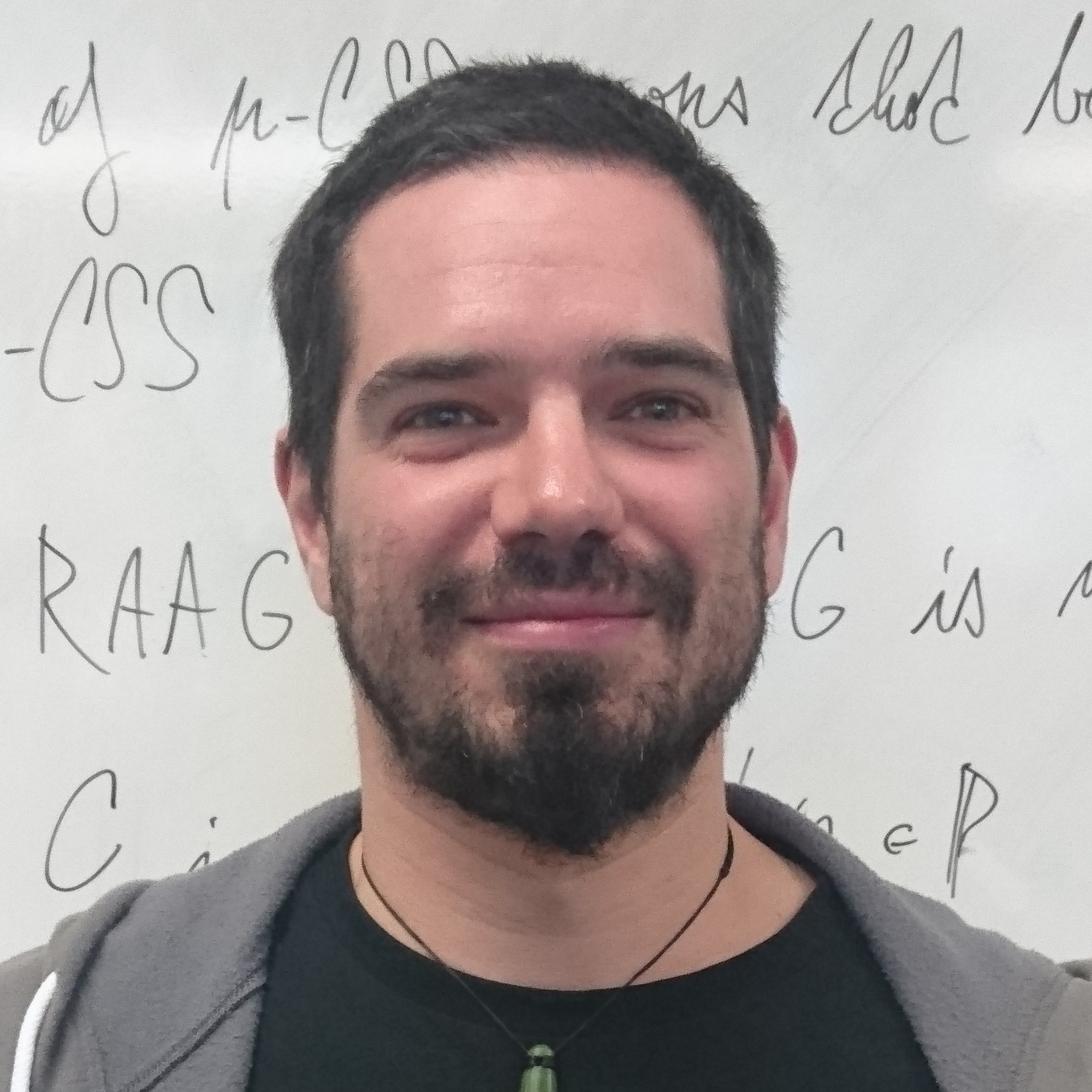Michal Ferov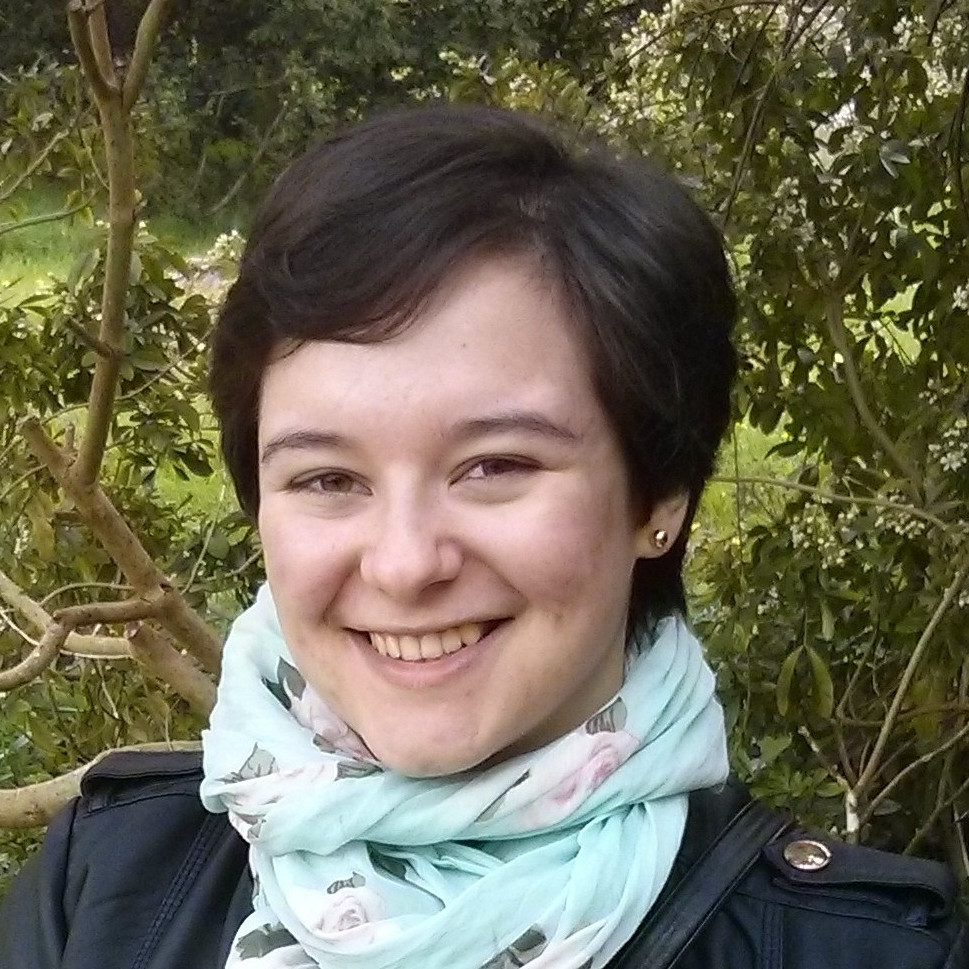Alejandra Garrido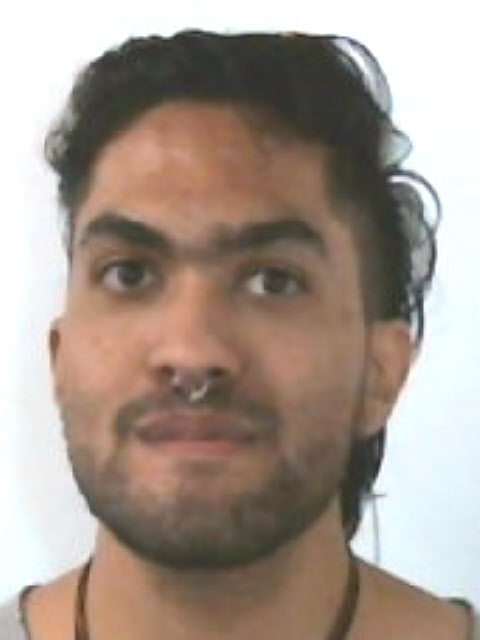João Vitor Pinto E Silva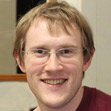Colin Reid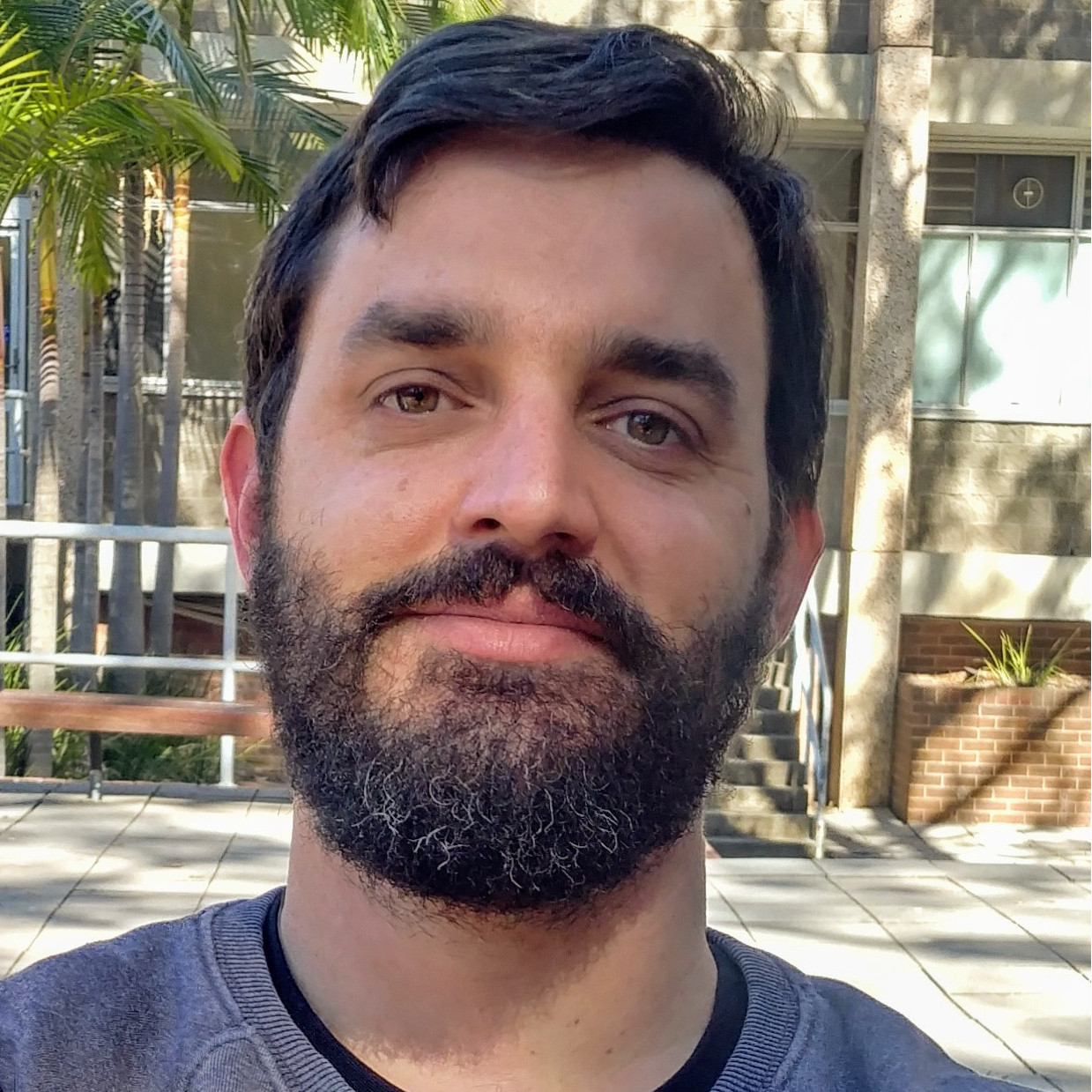Dave Robertson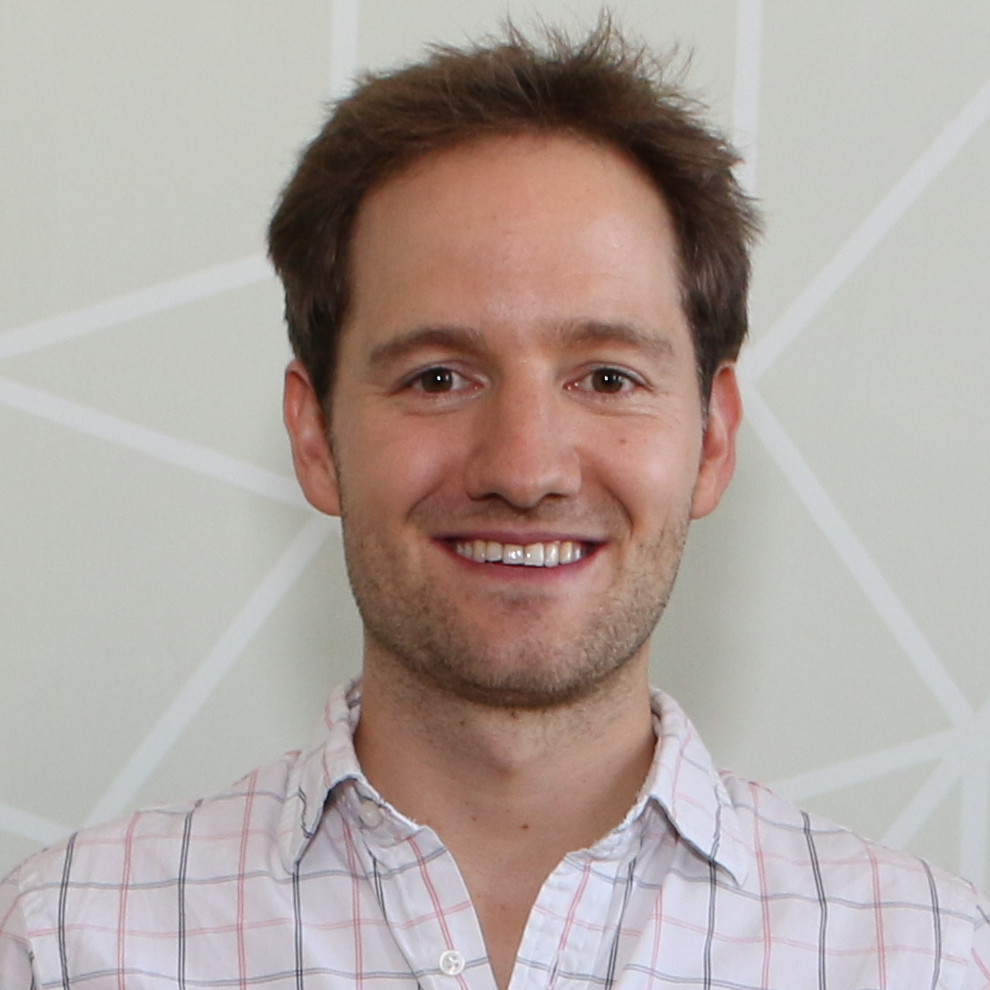Stephan Tornier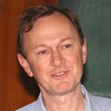George Willis ### October 2019 ## First-Year Summer Projects ### We offer six summer project scholarships around the topic "Puzzles, Codes and Groups" for first year students to be conducted in January and February 2020. Apply to juliane.turner@newcastle.edu.au with your student number. A flyer for the project can be found here. In this summer project you will explore the mathematical formalisation of the everyday notion of symmetry, which is the algebraic concept of a 'group'. Using groups, we are able to state with certainty whether a given puzzle can be solved (the 15-puzzle can not!) and, if so, compute how many steps are needed. As an example of the far-reaching applicability of this concept, we will look into error-correcting codes, such as the Golay code, which are critical in any digital communication, including NASA's Voyager program. Apart from theoretical studies in the area of groups, possible subprojects include the analysis of a given puzzle, the creation of new ones and the formulation of solution algorithms, as well as the design, analysis and implementation of codes. A team of up to six students will work on this project. ### September 2019 ## Summer Projects 2019/20 ### The following student research projects are related to the Australian Research Council project on 0-Dimensional Symmetry. While they are independent, each one gives a different view on the overall project. Another, more technical, description of this research is that it concerns totally disconnected, locally compact groups. An overview which attempts to explain the broader research project to non-experts may be seen here.  Symmetry of Graphs A graph (a network of vertices and edges) is \emph{vertex-transitive} if it 'looks' the same at all vertices. In algebraic terms, this means that, for any pair of vertices, there is an automorphism of the graph which maps the first vertex to the second. A necessary, but not sufficient, condition for a graph to be vertex-transitive is that all vertices should have the same valency. Analysing graph symmetry therefore involves a deeper study of the relationship between graphs and their automorphism groups than vertex valencies. Vertex-transitive graphs need not be edge-transitive, for example, the horizontal edges of a triangular pyramid lie on 3-cycles in the graph but the vertical edges do not. The particular question investigated in this project is how its symmetry group changes as edges are added to, or removed from, a graph. For finite graphs, a classical theorem of Burnside is relevant when the graph has prime order and, for infinite graphs, the goal is to reduce the vertex valency since this number controls important features of the symmetry group. Students taking this project will extend their knowledge of combinatorics and algebra, and how these two topics interact. The project will be jointly supervised by Brian Alspach. Solvable and nilpotent groups The notion of a solvable group originated with the work of É. Galois (1832), who showed that a polynomial equation has a solution by radicals if and only if its group of symmetries is solvable. For example, the formula$x=-b\pm\sqrt{b^{2}-4ac}/2a$is the solution of a quadratic equation by radicals and symmetries of the equation swap the$+$and$-$signs. (A group that is not solvable has some factors which are simple.) Groups of upper triangular$n\times n$real matrices are solvable and also have the topological property of being connected. It may be shown that these are essentially all the connected solvable groups. This project investigates solvable totally disconnected, locally compact groups. Our starting point is groups of upper triangular matrices having integer entries. These groups have the property of being nilpotent, which is stronger than solvability. Students taking this project will extend their knowledge of algebra, analysis and number theory. 'Totally disconnected' and 'locally compact' are topological notions; 'group', 'solvable and 'nilpotent' are algebraic ones; and the integer matrices embed into matrices over the real numbers as well as over other number fields. Symmetries of Rooted Trees Self-similar symmetry groups of rooted trees contribute to the study of totally disconnected, locally compact (or$0$-dimensional) groups in much the same way as eigenvalues and eigenvectors contribute to linear algebra. Whereas eigenvalues are complex numbers and we have a complete picture of what they all are, we are still at the stage of trying to describe self-similar symmetry groups of rooted trees. It is not necessary to understand the link with the theory of$0$-dimensional symmetry or with eigenvalues in order to describe these groups. This project aims to find an alternative geometric interpretation of some of the symmetry groups of trees. The idea being that describing these geometries might be a more natural approach to describing the groups. Computer algebra software will be used to analyse how the groups act on pairs, triples, etc. of vertices of the trees and then study the polyhedra in which these are edges, faces, etc. Observed patterns in the geometries may be able to be extrapolated to produce new families of groups. Students taking this project will extend their knowledge of algebra, graph theory and mathematical software. 'Group' is an algebraic notion and 'rooted tree' is a combinatorial one which arises in the study of data structures in computer science. 'Totally disconnected' and 'locally compact' are topological notions which, although relevant to the background, are not needed for this project. Visualising$0$-dimensional symmetry The word 'symmetry' brings to mind visual images and geometry. It has a broader meaning in mathematics, where we think of regularly repeating patterns and invariance under transformations as displaying symmetry, and where the language of algebra is used to describe symmetry. Visualising the patterns or the dynamics of the transformations remains an effective tool for understanding the algebra however. This project aims to develop software for visualising various aspects of$0$-dimensional symmetry, which is the symmetry of infinite networks and arises in number theory and other parts of algebra as well. The aim is to produce software which may be used by researchers and which will be made available on web-pages of the$0$-Dimensional Symmetry project. Students taking this project will extend their knowledge of algebra, analysis, mathematical software and coding skills. 'Totally disconnected' and 'locally compact' are topological notions; 'group' is an algebraic one; and other concepts will be met in the course of the project. Groups Acting on Trees: Compatible Local Actions The concept of symmetry is pervasive in mathematics and formalised in the algebraic notion of a 'group'. It is often natural to equip a group with a 'topology' - a generalisation of distance functions - which, in a sense, gives groups a shape. For example, the symmetry groups of infinite networks, or 'graphs', become zero-dimensional. A versatile and accessible class of these groups was defined by Burger-Mozes and refined by this project's supervisor: Picture an infinite graph in which every vertex has the same number of neighbours and consider only those symmetries of the graph which in a neighbourhood of every given vertex act like one of finitely many allowed 'local actions'. In order for the resulting family of symmetries to reflect these restrictions accurately, the local actions need to satisfy certain conditions. The aim of this project is to find more general constructions of such local actions and use them to test the sharpness of an existing rigidity theorem. A student who takes this project will extend his/her knowledge of algebra and learn how to use computer algebra systems designed for computations in group theory, including coding skills. Groups Acting on Trees without Involutive Inversions The concept of symmetry is pervasive in mathematics and formalised in the algebraic notion of a 'group'. It is often natural to equip a group with a 'topology' - a generalisation of distance functions - which, in a sense, gives groups a shape. For example, the symmetry groups of infinite networks, or 'graphs', become zero-dimensional. A versatile and accessible class of these groups was defined by Burger-Mozes and refined by this project's supervisor: Picture an infinite graph in which every vertex has the same number of neighbours and consider only those symmetries of the graph which in a neighbourhood of every given vertex act like one of finitely many allowed 'local actions'. In order for the resulting family of symmetries to reflect these restrictions accurately, the local actions need to satisfy certain conditions. The aim of this project is to define a new class of groups acting on said graph by restricting the local action on edge neighbourhoods rather than vertex neighbourhoods, and thereby gain a new perspective on existing examples of graph symmetry groups relating to the Weiss conjecture. A student who takes this project will extend his/her knowledge of algebra and topology, and develop proofs intertwining both. ### April 2019 ## Special Semester at the Bernoulli Center ### Our research group was recently awarded a special semester at the Bernoulli Center in Lausanne, Switzerland. The semester entitled "Locally compact groups acting on discrete structures" is to take place in the second half of 2020 and will comprise a summer school, several workshops as well as special lectures, bringing together researchers in the field across all academic levels.### November 2018 ## PhD Scholarship ### A PhD scholarship opportunity is available for students to investigate totally disconnected, locally compact groups under the supervision of ARC Laureate Professor George Willis. The students will join a team seeking to bring our understanding of these groups to a level comparable to that of finite. ### October 2018 ## Summer Projects 2018/19 ### The following student research projects are related to the Australian Research Council project on 0-Dimensional Symmetry. While they are independent, each one gives a different view on the overall project. Another, more technical, description of this research is that it concerns totally disconnected, locally compact groups. An overview which attempts to explain the broader research project to non-experts may be seen here.  Simple groups of infinite matrices An essential step towards understanding$0$-dimensional symmetry is to describe the totally disconnected, locally compact (t.d.l.c.) groups which are simple. Simple groups are those which cannot be factored into smaller pieces and they are sometimes called the 'atoms of symmetry', or said to be analogues of the prime numbers in number theory. This project investigates t.d.l.c. groups of infinite matrices. It is suspected that these groups will be found to be simple and we will aim to show that by first studying corresponding groups of$n\times n$matrices which are known to be simple. Students taking this project will extend their knowledge of algebra, analysis and number theory. 'Totally disconnected', 'locally compact' and '$0$-dimensional' are topological notions; 'group' and 'simple' are algebraic ones; and the matrix entries are numbers modulo a prime number$p$. Solvable and nilpotent groups The notion of a solvable group originated with the work of É. Galois (1832), who showed that a polynomial equation has a solution by radicals if and only if its group of symmetries is solvable. For example, the formula$x=-b\pm\sqrt{b^{2}-4ac}/2a$is the solution of a quadratic equation by radicals and symmetries of the equation swap the$+$and$-$signs. (A group that is not solvable has some factors which are simple.) Groups of upper triangular$n\times n$real matrices are solvable and also have the topological property of being connected. It may be shown that these are essentially all the connected solvable groups. This project investigates solvable totally disconnected, locally compact groups. Our starting point is groups of upper triangular matrices having integer entries. These groups have the property of being nilpotent, which is stronger than solvability. Students taking this project will extend their knowledge of algebra, analysis and number theory. 'Totally disconnected' and 'locally compact' are topological notions; 'group', 'solvable and 'nilpotent' are algebraic ones; and the integer matrices embed into matrices over the real numbers as well as over other number fields. Free products of graphs Symmetries of networks (or graphs) are '$0$-dimensional', and such symmetries are investigated through the algebraic technique of totally disconnected, locally compact groups. We are interested in highly symmetric, infinite graphs and one way to form such graphs is by gluing together infinitely many copies of finite graphs according to some regular instructions. This project investigates the symmetry groups of examples of graphs formed in this way and compares them with the symmetry groups of infinite regular trees, which are the most basic type of infinite regular graph. The aim is to determine whether the symmetry groups obtained in this way are simple and new. Students taking this project will extend their knowledge of algebra, analysis and combinatorics. 'Totally disconnected' and 'locally compact' are topological notions; 'group' and 'simple' are algebraic ones; and 'graphs' are a combinatorial concept. Visualising$0$-dimensional symmetry The word 'symmetry' brings to mind visual images and geometry. It has a broader meaning in mathematics, where we think of regularly repeating patterns and invariance under transformations as displaying symmetry, and where the language of algebra is used to describe symmetry. Visualising the patterns or the dynamics of the transformations remains an effective tool for understanding the algebra however. This project aims to develop software for visualising various aspects of$0$-dimensional symmetry, which is the symmetry of infinite networks and arises in number theory and other parts of algebra as well. The aim is to produce software which may be used by researchers and which will be made available on web-pages of the$0$-Dimensional Symmetry project. Students taking this project will extend their knowledge of algebra, analysis, mathematical software and coding skills. 'Totally disconnected' and 'locally compact' are topological notions; 'group' is an algebraic one; and other concepts will be met in the course of the project. ### December 2019 - February 2020 ## Summer Project: Groups acting on trees without involutive inversions ### Jack Berry The concept of symmetry is pervasive in mathematics and formalised in the algebraic notion of a 'group'. It is often natural to equip a group with a 'topology' - a generalisation of distance functions - which, in a sense, gives groups a shape. For example, the symmetry groups of infinite networks, or 'graphs', become zero-dimensional. A versatile and accessible class of these groups was defined by Burger-Mozes and refined by this project's supervisor: Picture an infinite graph in which every vertex has the same number of neighbours and consider only those symmetries of the graph which in a neighbourhood of every given vertex act like one of finitely many allowed 'local actions'. In order for the resulting family of symmetries to reflect these restrictions accurately, the local actions need to satisfy certain conditions. The aim of this project is to define a new class of groups acting on said graph by restricting the local action on edge neighbourhoods rather than vertex neighbourhoods, and thereby gain a new perspective on existing examples of graph symmetry groups relating to the Weiss conjecture. A student who takes this project will extend his/her knowledge of algebra and topology, and develop proofs intertwining both. ### July 2019 - September 2019 ## Winter Project: Visualisations of buildings ### Tasman Fell### December 2018 - February 2019 ## Summer Project: Simple groups of infinite matrices ### Peter Groenhout An essential step towards understanding$0$-dimensional symmetry is to describe the totally disconnected, locally compact (t.d.l.c.) groups which are simple. Simple groups are those which cannot be factored into smaller pieces and they are sometimes called the 'atoms of symmetry', or said to be analogues of the prime numbers in number theory. This project investigates t.d.l.c. groups of infinite matrices. It is suspected that these groups will be found to be simple and we will aim to show that by first studying corresponding groups of$n\times n$matrices which are known to be simple. Students taking this project will extend their knowledge of algebra, analysis and number theory. 'Totally disconnected', 'locally compact' and '$0$-dimensional' are topological notions; 'group' and 'simple' are algebraic ones; and the matrix entries are numbers modulo a prime number$p$. Continuing work on this project resulted in a preprint which can be found here. ### December 2018 - February 2019 ## Summer Project: Free products of graphs ### Max Carter Symmetries of networks (or graphs) are '$0$-dimensional', and such symmetries are investigated through the algebraic technique of totally disconnected, locally compact groups. We are interested in highly symmetric, infinite graphs and one way to form such graphs is by gluing together infinitely many copies of finite graphs according to some regular instructions. This project investigates the symmetry groups of examples of graphs formed in this way and compares them with the symmetry groups of infinite regular trees, which are the most basic type of infinite regular graph. The aim is to determine whether the symmetry groups obtained in this way are simple and new. Students taking this project will extend their knowledge of algebra, analysis and combinatorics. 'Totally disconnected' and 'locally compact' are topological notions; 'group' and 'simple' are algebraic ones; and 'graphs' are a combinatorial concept. ### December 2018 - February 2019 ## Summer Project: Random Walks on Derived Graphs ### Alastair Anderberg A simple random walk through$\mathbb{Z}^{d}$describes a path taken through a d-dimensional integer lattice, where each of the 2d possible directions is chosen with equal probability. In 1921, George Polya proved that$d=1$or$d=2$dimensions, such a path will return to its starting position almost surely, but for$d=3$dimensions or higher, the probability of returning to the the starting position decreases as the number of dimensions increases. In this project, we plan to generalise this idea from the integer lattice to more complicated structures through the idea of derived graphs. # Symmetry in Newcastle (SIN) This is a series of meetings with the aim of bringing together mathematicians working on Symmetry - broadly understood - that are based around Newcastle - also broadly understood. Topics of interest include all aspects of group theory and connections to computer science, dynamics, graph theory, logic, number theory, operator algebras, topology. All interested are warmly invited to attend. It's also a great opportunity to discover why Newcastle is Australia's coolest coastal town! The mailing list carries updates and reminders as new seminars are announced. To be added to or removed from the mailing list, or for any other information, please contact Alejandra Garrido. Getting there: The venue (search for room) on the Callaghan campus of The University of Newcastle can be reached in at least three ways: By bus, going to "Mathematics Building, Ring Rd"; by train, going to "Warabrook Station" and walking about 15-20 minutes across the campus; or by car, parking e.g. in carpark "P2".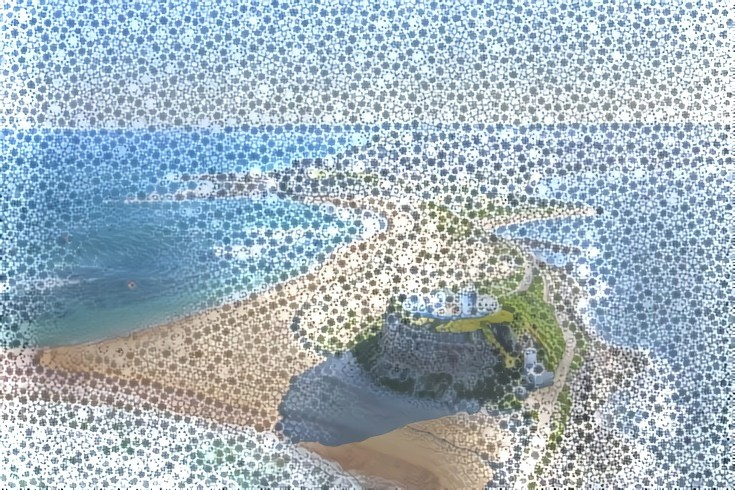## Upcoming Events Date Time Room Speaker Title TBA 12.00-13.00 TBA TBA TBA 14.00-15.00 TBA TBA TBA 15.30-16.30 TBA TBA TBA ## Past Events  01.11.2019 12.00-13.00 V 109 Anthony Dooley Classification of non-singular systems and critical dimension A non-singular measurable dynamical system is a measure space$X$whose measure$\mu$has the property that$\mu $and$\mu \circ T$are equivalent measures (in the sense that they have the same sets of measure zero). Here$T$is a bimeasurable invertible transformation of$X$. The basic building blocks are the \emph{ergodic} measures. Von Neumann proposed a classification of non-singular ergodic dynamical systems, and this has been elaborated subsequently by Krieger, Connes and others. This work has deep connections with C*-algebras. I will describe some work of myself, collaborators and students which explore the classification of dynamical systems from the point of view of measure theory. In particular, we have recently been exploring the notion of critical dimension, a study of the rate of growth of sums of Radon-Nikodym derivatives$\Sigma_{k=1}^n \frac{d\mu \circ T^k}{d\mu}$. Recently, we have been replacing the single transformation$T$with a group acting on the space$X$. 14.00-15.00 V 109 Colin Reid Piecewise powers of a minimal homeomorphism of the Cantor set Let$X$be the Cantor set and let$g$be a minimal homeomorphism of$X$(that is, every orbit is dense). Then the topological full group$\tau[g]$of$g$consists of all homeomorphisms$h$of$X$that act 'piecewise' as powers of$g$, in other words,$X$can be partitioned into finitely many clopen pieces$X_1,...,X_n$such that for each$i$,$h$acts on$X_i$as a constant power of$g$. Such groups have attracted considerable interest in dynamical systems and group theory, for instance they characterize the homeomorphism up to flip conjugacy (Giordano-Putnam-Skau) and they provided the first known examples of infinite finitely generated simple amenable groups (Juschenko--Monod). My talk is motivated by the following question: given$h\in\tau[g]$for some minimal homeomorphism$g$, what can the closures of orbits of$h$look like? Certainly$h\in\tau[g]$is not minimal in general, but it turns out to be quite close to being minimal, in the following sense: there is a decomposition of$X$into finitely many clopen invariant pieces, such that on each piece$h$acts a homeomorphism that is either minimal or of finite order. Moreover, on each of the minimal parts of$h$, then either$h$or$h^{-1}$has a 'positive drift' with respect to the orbits of$g$; in fact, it can be written in a canonical way as a conjugate of a product of induced transformations (aka first return maps) of$g$. No background knowledge of topological full groups is required; I will introduce all the necessary concepts in the talk. 15.30-16.30 V 109 Michael Barnsley Dynamics on Fractals I will outline a new theory of fractal tilings. The approach uses graph iterated function systems (IFS) and centers on underlying symbolic shift spaces. These provide a zero dimensional representation of the intricate relationship between shift dynamics on fractals and renormalization dynamics on spaces of tilings. The ideas I will describe unify, simplify, and substantially extend key concepts in foundational papers by Solomyak, Anderson and Putnam, and others. In effect, IFS theory on the one hand, and self-similar tiling theory on the other, are unified. The work presented is largely new and has not yet been submitted for publication. It is joint work with Andrew Vince (UFL) and Louisa Barnsley. The presentation will include links to detailed notes. The figures illustrate 2d fractal tilings. By way of recommended background reading I mention the following awardwinning paper: M. F. Barnsley, A. Vince, Self-similar polygonal tilings, Amer. Math. Monthly 124 (1017) 905-921.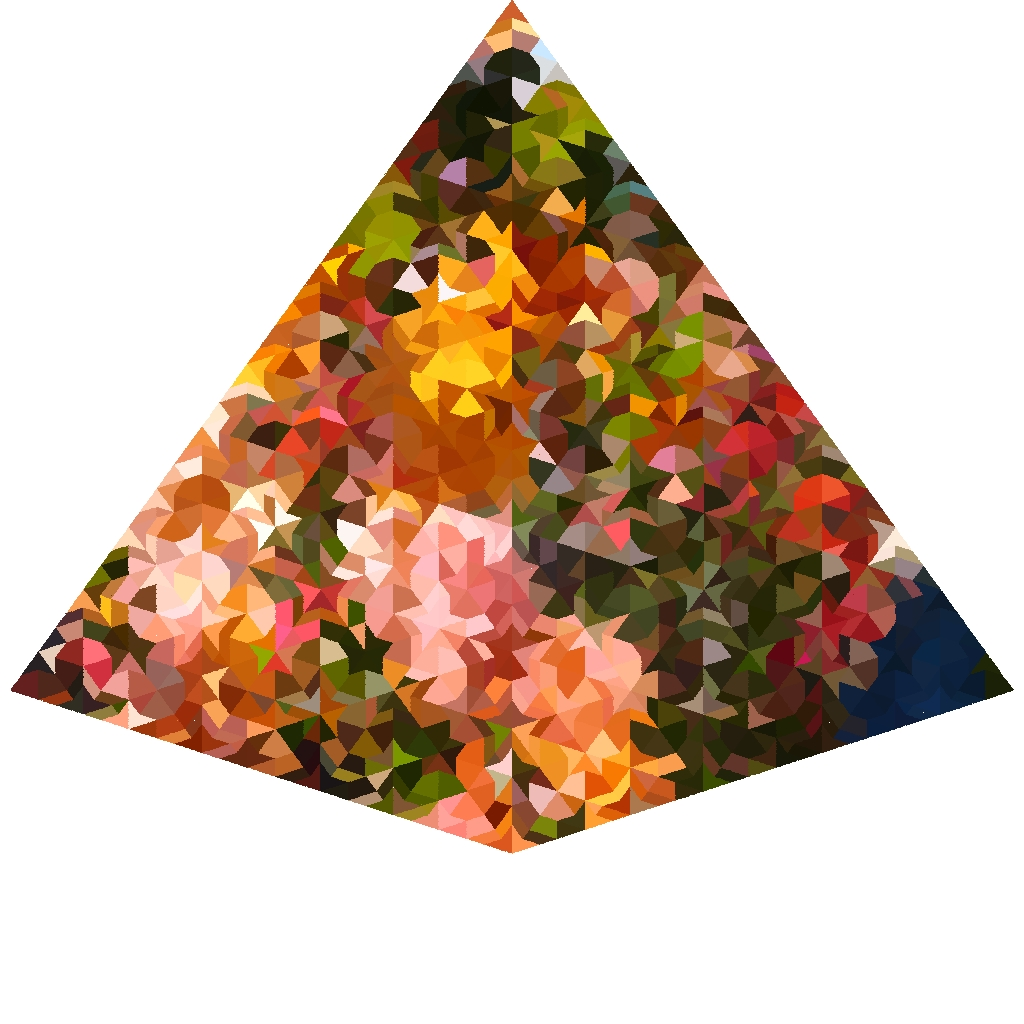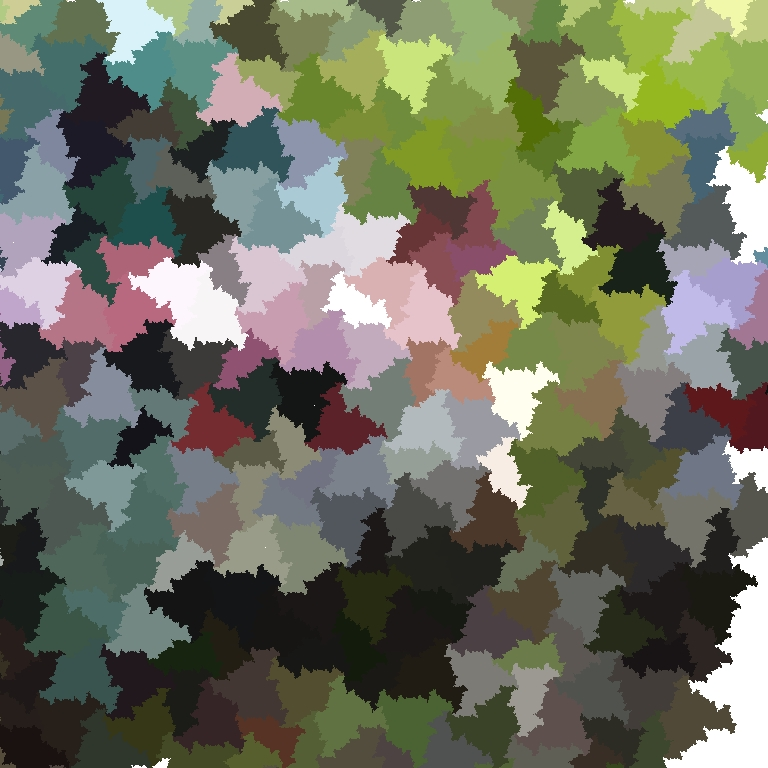06.09.2019 14.30-15.30 V 109 Sasha Fish Patterns in sets of positive density in trees and buildings We will discuss what are the patterns that necessarily occur in sets of positive density in homogeneous trees and certain affine buildings. Based on joint work with Michael Bjorklund (Chalmers) and James Parkinson (University of Sydney). 16.00-17.00 V 109 Michael Coons Integer sequences, asymptotics and diffraction For some time now, I have been trying to understand the intricacy and complexity of integer sequences from a variety of different viewpoints and at least at some level trying to reconcile these viewpoints. However vague that sounds - and it certainly is vague to me - in this talk I hope to explain this sentiment a bit. While a variety of results will be considered, I will focus closely on two examples of wider interest, the Thue-Morse sequence and the set of$k$-free integers. 02.08.2019 12.00-13.00 V 109 Brian Alspach Honeycomb Toroidal Graphs The honeycomb toroidal graphs are a family of graphs I have been looking at now and then for thirty years. I shall discuss an ongoing project dealing with hamiltonicity as well as some of their properties which have recently interested the computer architecture community. 14.00-15.00 V 109 John Bamberg Symmetric finite generalised polygons Finite generalised polygons are the rank 2 irreducible spherical buildings, and include projective planes and the generalised quadrangles, hexagons, and octagons. Since the early work of Ostrom and Wagner on the automorphism groups of finite projective planes, there has been great interest in what the automorphism groups of generalised polygons can be, and in particular, whether it is possible to classify generalised polygons with a prescribed symmetry condition. For example, the finite Moufang polygons are the 'classical' examples by a theorem of Fong and Seitz (1973-1974) (and the infinite examples were classified in the work of Tits and Weiss (2002)). In this talk, we give an overview of some recent results on the study of symmetric finite generalised polygons, and in particular, on the work of the speaker with Cai Heng Li and Eric Swartz. 15.30-16.30 V 109 Marston Conder Edge-transitive graphs and maps In this talk I'll describe some recent discoveries about edge-transitive graphs and edge-transitive maps. These are objects that have received relatively little attention compared with their vertex-transitive and arc-transitive siblings. First I will explain a new approach (taken in joint work with Gabriel Verret) to finding all edge-transitive graphs of small order, using single and double actions of transitive permutation groups. This has resulted in the determination of all edge-transitive graphs of order up to 47 (the best possible just now, because the transitive groups of degree 48 are not known), and bipartite edge-transitive graphs of order up to 63. It also led us to the answer to a 1967 question by Folkman about the valency-to-order ratio for regular graphs that are edge- but not vertex-transitive. Then I'll describe some recent work on edge-transitive maps, helped along by workshops at Oaxaca and Banff in 2017. I'll explain how such maps fall into 14 natural classes (two of which are the classes of regular and chiral maps), and how graphs in each class may be constructed and analysed. This will include the answers to some 18-year-old questions by Širáň, Tucker and Watkins about the existence of particular kinds of such maps on orientable and non-orientable surfaces. 03.05.2019 14.00-15.00 W 238 Heiko Dietrich Quotient algorithms (a.k.a. how to compute with finitely presented groups) In this talk, I will survey some of the famous quotient algorithms that can be used to compute efficiently with finitely presented groups. The last part of the talk will be about joint work with Alexander Hulpke (Colorado State University): we have looked at quotient algorithms for non-solvable groups, and I will report on the findings so far. 15.30-16.30 W 238 Youming Qiao Isomorphism testing problems: in light of Babai's graph isomorphism breakthrough In computer science, an isomorphism testing problem asks whether two objects are in the same orbit under a group action. The most famous problem of this type has been the graph isomorphism problem. In late 2015, L. Babai announced a quasipolynomial-time algorithm for the graph isomorphism problem, which is widely regarded as a breakthrough in theoretical computer science. This leads to a natural question, that is, which isomorphism testing problems should naturally draw our attention for further exploration? 12.00-13.00 W 238 Nicole Sutherland Computations of Galois groups and splitting fields The Galois group of a polynomial is the automorphism group of its splitting field. These automorphisms act by permuting the roots of the polynomial so that a Galois group will be a subgroup of a symmetric group. Using the Galois group the splitting field of a polynomial can be computed more efficiently than otherwise, using the knowledge of the symmetries of the roots. I will present an algorithm developed by Fieker and Klueners, which I have extended, for computing Galois groups of polynomials over arithmetic fields as well as approaches to computing splitting fields using the symmetries of the roots. 05.04.2019 12.00-13.00 W 238 Arnaud Brothier Jones' actions of the Thompson's groups: applications to group theory and mathematical physics Motivating in constructing conformal field theories Jones recently discovered a very general process that produces actions of the Thompson groups$F$,$T$and$V$such as unitary representations or actions on$C^{\ast}$-algebras. I will give a general panorama of this construction along with many examples and present various applications regarding analytical properties of groups and, if time permits, in lattice theory (e.g. quantum field theory). 14.00-15.00 W 238 Lawrence Reeves An irrational-slope Thompson's group Let$t$be the the multiplicative inverse of the golden mean. In 1995 Sean Cleary introduced the irrational-slope Thompson's group$F_t$, which is the group of piecewise-linear maps of the interval$[0,1]$with breaks in$Z[t]$and slopes powers of$t$. In this talk we describe this group using tree-pair diagrams, and then demonstrate a finite presentation, a normal form, and prove that its commutator subgroup is simple. This group is the first example of a group of piecewise-linear maps of the interval whose abelianisation has torsion, and it is an open problem whether this group is a subgroup of Thompson's group$F$. 15.30-16.30 W 238 Richard Garner Topos-theoretic aspects of self-similarity A Jonsson-Tarski algebra is a set$X$endowed with an isomorphism$X\to XxX$. As observed by Freyd, the category of Jonsson-Tarski algebras is a Grothendieck topos - a highly structured mathematical object which is at once a generalised topological space, and a generalised universe of sets. In particular, one can do algebra, topology and functional analysis inside the Jonsson-Tarski topos, and on doing so, the following objects simply pop out: Cantor space; Thompson's group V; the Leavitt algebra L2; the Cuntz semigroup S2; and the reduced$C^{\ast}$-algebra of S2. The first objective of this talk is to explain how this happens. The second objective is to describe other "self-similar toposes" associated to, for example, self-similar group actions, directed graphs and higher-rank graphs; and again, each such topos contains within it a familiar menagerie of algebraic-analytic objects. If time permits, I will also explain a further intriguing example which gives rise to Thompson's group F and, I suspect, the Farey AF algebra. No expertise in topos theory is required; such background as is necessary will be developed in the talk. In this edition, three 2017 ARC Laureate Fellows in mathematics outline their projects. Descriptions of these projects are given as abstracts below.  15.03.2019 12.00-13.00 Purdue Room Mathai Varghese Advances in Index Theory The project aims to develop novel techniques to investigate Geometric analysis on infinite dimensional bundles, as well as Geometric analysis of pathological spaces with Cantor set as fibre, that arise in models for the fractional quantum Hall effect and topological matter, areas recognised with the 1998 and 2016 Nobel Prizes. Building on the applicant's expertise in the area, the project will involve postgraduate and postdoctoral training in order to enhance Australia's position at the forefront of international research in Geometric Analysis. Ultimately, the project will enhance Australia's leading position in the area of Index Theory by developing novel techniques to solve challenging conjectures, and mentoring HDR students and ECRs. 14.00-15.00 Purdue Room Fedor Sukochev Breakthrough methods for noncommutative calculus This project aims to solve hard, outstanding problems which have impeded our ability to progress in the area of quantum or noncommutative calculus. Calculus has provided an invaluable tool to science, enabling scientific and technological revolutions throughout the past two centuries. The project will initiate a program of collaboration among top mathematical researchers from around the world and bring together two separate mathematical areas into a powerful new set of tools. The outcomes from the project will impact research at the forefront of mathematical physics and other sciences and enhance Australia's reputation and standing. 15.30-16.30 Purdue Room George Willis Zero-Dimensional Symmetry and its Ramifications This project aims to investigate algebraic objects known as 0-dimensional groups, which are a mathematical tool for analysing the symmetry of infinite networks. Group theory has been used to classify possible types of symmetry in various contexts for nearly two centuries now, and 0-dimensional groups are the current frontier of knowledge. The expected outcome of the project is that the understanding of the abstract groups will be substantially advanced, and that this understanding will shed light on structures possessing 0-dimensional symmetry. In addition to being cultural achievements in their own right, advances in group theory such as this also often have significant translational benefits. This will provide benefits such as the creation of tools relevant to information science and researchers trained in the use of these tools. 01.03.2019 12.00-13.00 W 104 Marcelo Laca An introduction to KMS states and two suprising examples The KMS condition for equilibrium states of C*-dynamical systems has been around since the 1960's. With the introduction of systems arising from number theory and from semigroup dynamics following pioneering work of Bost and Connes, their study has accelerated significantly in the last 25 years. I will give a brief introduction to C*-dynamical systems and their KMS states and discuss two constructions that exhibit fascinating connections with key open questions in mathematics such as Hilbert's 12th problem on explicit class field theory and Furstenberg's x2 x3 conjecture. 14.00-15.00 W 104 Zahra Afsar KMS states of$C^*$-algebras of$*$-commuting local homeomorphisms and applications in$k$-graph algebras In this talk, I will show how to build$C^*$-algebras using a family of local homeomorphisms. Then we will compute the KMS states of the resulted algebras using Laca-Neshveyev machinery. Then I will apply this result to$C^*$-algebras of$K$-graphs and obtain interesting$C^*$-algebraic information about$k$-graph algebras. This talk is based on a joint work with Astrid an Huef and Iain Raeburn. 15.30-16.30 W 104 Aidan Sims What equilibrium states KMS states for self-similar actions have to do with fixed-point theory Using a variant of the Laca-Raeburn program for calculating KMS states, Laca, Raeburn, Ramagge and Whittaker showed that, at any inverse temperature above a critical value, the KMS states arising from self-similar actions of groups (or groupoids)$G$are parameterised by traces on C*(G). The parameterisation takes the form of a self-mapping$\chi$of the trace space of C*(G) that is built from the structure of the stabilisers of the self-similar action. I will outline how this works, and then sketch how to see that$\chi\$ has a unique fixed-point, which picks out the preferred" trace of C*(G) corresponding to the only KMS state that persists at the critical inverse temperature. The first part of this will be an exposition of results of Laca-Raeburn-Ramagge-Whittaker. The second part is joint work with Joan Claramunt.
Date Time Room Speaker Title
18.11.2019 10.00 V 108 TBA

11.11.2019 10.00 V 108 Buildings: Strongly transitive automorphism groups

04.11.2019 10.00 V 108 Buildings: Strongly transitive automorphism groups

28.10.2019 10.00 V 108 Buildings: Retractions and apartment systems

21.10.2019 10.00 V 108 Buildings: Flag complexes of incidence geometries

14.10.2019 10.00 V 108 Buildings: Definition and First Properties

08.10.2019 10.00 SR 118 Thin chamber complexes

30.09.2019 10.00 V 108 Endomorphisms of Coxeter Complexes

23.09.2019 10.00 V 108 Coxeter Complexes

16.09.2019 10.00 V 108 Abstract Reflection Groups: Equivalent Conditions

02.09.2019 10.00 V 108 Examples

29.08.2019 10.00 V 108 Abstract Reflection Groups: In Search of Axioms

26.08.2019 10.00 V 102 The simplicial complex associated to a reflection groups

22.08.2019 10.00 V 108 Cell decomposition

12.08.2019 10.00 V 108 Alejandra Garrido Root systems

08.08.2019 10.00 V 102 Alejandra Garrido Root systems and Dynkin diagrams

05.08.2019 11.00 V 108 Alejandra Garrido Root systems and finite reflection groups

22.05.2019 10.00 W 243 Dave Robertson Essentially Chief Series

06.05.2019 13.00 W 243 Dave Robertson Essentially Chief Series

01.05.2019 10.00 W 243 Dave Robertson Essentially Chief Series

29.04.2019 13.00 W 243 Dave Robertson Essentially Chief Series

10.04.2019 10.00 W 243 Dave Robertson Essentially Chief Series

08.04.2019 13.00 W 243 Dave Robertson Essentially Chief Series

01.04.2019 13.00 W 243 Stephan Tornier Cayley-Abels graphs

20.03.2019 10.00 W 243 Stephan Tornier Cayley-Abels graphs

18.03.2019 13.00 W 243 Alejandra Garrido Cayley-Abels graphs

13.03.2019 10.00 W 243 Alejandra Garrido Cayley-Abels graphs

11.03.2019 13.00 W 243 Alejandra Garrido Cayley-Abels graphs

06.03.2019 10.00 W 243 Alejandra Garrido Cayley-Abels graphs

04.03.2019 13.00 W 243 Michal Ferov Cayley-Abels graphs

27.02.2019 10.00 W 243 Michal Ferov Cayley-Abels graphs

25.02.2019 13.00 W 243 Michal Ferov Cayley-Abels graphs

20.02.2019 13.00 SR 118 Michal Ferov Cayley-Abels graphs

17.12.2018 14.00 MC G29 Stephan Tornier Haar measures

10.12.2018 14.00 MC G29 Dave Robertson Haar measures

04.12.2018 all week Adelaide AustMS Meeting

03.12.2018 all week Adelaide AustMS Meeting

27.11.2018 9.00 MC G29 Dave Robertson Haar measures

26.11.2018 14.00 MC G29 CARMA Retreat

20.11.2018 9.00 MC G29 Dave Robertson Haar measures

19.11.2018 14.00 MC G29 Dave Robertson Haar measures

13.11.2018 all day NeW Space EViMS Workshop

12.11.2018 Seminar

06.11.2018 all day Sydney Group Actions Seminar held at the University of Sydney

05.11.2018 14.00 MC G29 Exercise Session

30.10.2018 14.00 MC G29 Stephan Tornier Semidirect products and restricted direct products

30.10.2018 9.00 MC G29 Michal Ferov Locally finite graphs

29.10.2018 14.00 MC G29 Michal Ferov Locally finite graphs

22.10.2018 14.00 MC G29 Michal Ferov Topological Isomorphism Theorems

16.10.2018 9.00 MC G29 Michal Ferov Topological structure of t.d.l.c. Polish groups

15.10.2018 14.00 MC G29 Michal Ferov Van Dantzig's theorem

## Upcoming Events

Dates Place Event Participants
December 8-11, 2020 Armidale,
Australia
Meeting of the Australian Mathematical Society

July-December, 2020 Lausanne,
Switzerland
Locally compact acting on discrete structures Alejandra Garrido, Colin Reid, Stephan Tornier, George Willis

June 22-26, 2020 Edinburgh,
Scotland
Geometric and Asymptotic Group Theory
with Applications

June 21-27, 2020 Oberwolfach,
Germany
Geometric Structures in Group Theory

June 15-18, 2020 Paris,
France
Groups, Random Walks and Dynamics

February 10-14, 2020 Rotorua,
New Zealand
Symmetries of Discrete Objects

December 3-6, 2019 Melbourne,
Australia
Meeting of the Australian Mathematical Society Colin Reid

November 30 - December 1, 2019 Melbourne,
Australia
Australian Algebra Conference Colin Reid

## Past Events

 October 21-25, 2019 Providence RI, United States of America Illustrating Number Theory and Algebra Michal Ferov September 30 - October 4, 2019 Adelaide, Australia Analysis on Manifolds George Willis August 5-9, 2019 Sydney, Australia Flags, Galleries and Reflection Groups May 26-30, 2019 Tel Aviv, Israel Geometric and Asymptotic Group Theory with Applications Alejandra Garrido, Stephan Tornier April-July, 2019 Będlewo, Poland Geometric and Analytic Group Theory Alejandra Garrido March 24-29, 2019 Dagstuhl, Germany Algorithmic Problems in Group Theory Michal Ferov January 22-25, 2019 Zurich, Switzerland Groups, spaces, and geometries on the occasion of Alessandra Iozzi's 60th birthday Colin Reid, Stephan Tornier January 21-25, 2019 Sydney, Australia The Asia-Australia Algebra Conference 2019 Michal Ferov, Alejandra Garrido, George Willis January 16-18, 2019 Auckland, New Zealand Groups and Geometries January 11, 2019 London, England Geometric Group Theory meeting at Royal Holloway Colin Reid December 4-7, 2018 Adelaide, Australia Meeting of the Australian Mathematical Society Alejandra Garrido, Colin Reid, Dave Robertson, Stephan Tornier, George Willis November 14-16, 2018 Newcastle, Australia AMSI-CARMA workshop on Mathematical Thinking George Willis November 13, 2018 Newcastle, Australia Effective Visualisation in the Mathematical Sciences Alejandra Garrido, Colin Reid, Dave Robertson, Stephan Tornier, George Willis November 9-11, 2018 Newcastle, Australia Diagrammatic Reasoning in Higher Education Dave Robertson, Stephan Tornier September-December, 2018 Bonn, Germany Logic and Algorithms in Group Theory Michal Ferov, George Willis September 21, 2018 London, England Hausdorff Dimension Alejandra Garrido September 11-13, 2018 Geneva, Switzerland Spectra and L2 - invariants Alejandra Garrido September 3-7, 2018 Oxford, England Groups, Geometry and Representations Alejandra Garrido June 25-29, 2018 Düsseldorf, Germany Trees, dynamics and locally compact groups Michal Ferov, Alejandra Garrido, Colin Reid, Stephan Tornier, George Willis June 11-14, 2018 St. Andrews, England British Mathematical Colloquium Colin Reid January 23-26, 2018 Auckland, New Zealand Groups and Geometry Michal Ferov, Colin Reid, George Willis

The Group Actions Seminar held regularly at The University of Sydney

The Geometry and Topology Seminar held regularly at The University of Sydney